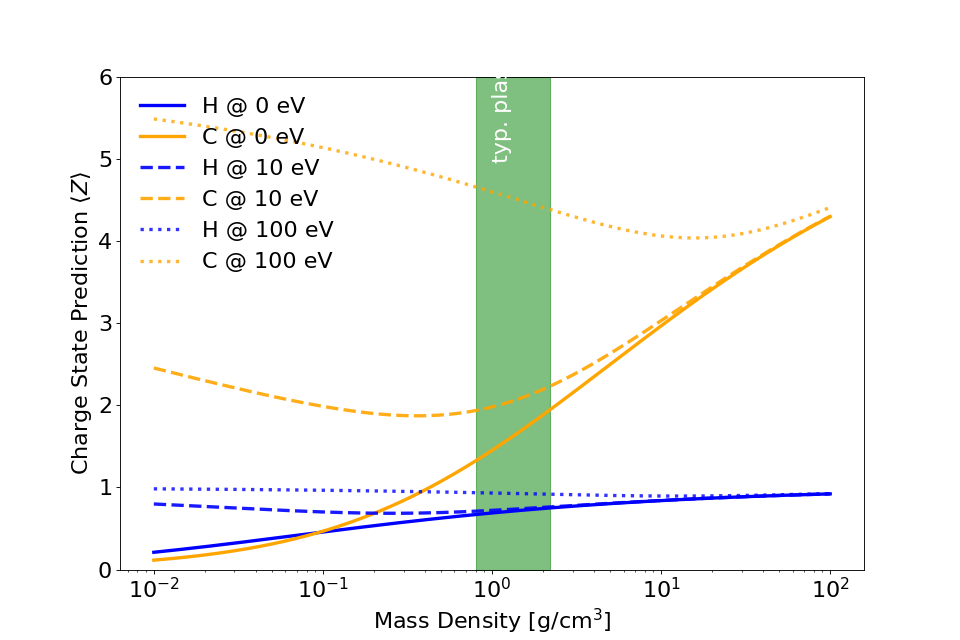# Collisional Ionization¶

## LTE Models¶

Module author: Marco Garten

Implemented LTE Model: Thomas-Fermi Ionization according to [More1985]

The implementation of the Thomas-Fermi model takes the following input quantities.

• ion proton number $$Z$$

• ion species mass density $$\rho$$

• electron “temperature” $$T$$

Due to the nature of our simulated setups it is also used in non-equilibrium situations. We therefore implemented additional conditions to mediate unphysical behavior but introduce arbitrariness.Here is an example of hydrogen (in blue) and carbon (in orange) that we would use in a compound plastic target, for instance. The typical plastic density region is marked in green. Two of the artifacts can be seen in this plot:

1. Carbon is predicted to have an initial charge state $$\langle Z \rangle > 0$$ even at $$T = 0\,\mathrm{eV}$$.

2. Carbon is predicted to have a charge state of $$\langle Z \rangle \approx 2$$ at solid plastic density and electron temperature of $$T = 10\,\mathrm{eV}$$ which increases even as the density decreases. The average electron kinetic energy at such a temperature is 6.67 eV which is less than the 24.4 eV of binding energy for that state. The increase in charge state with decreasing density would lead to very high charge states in the pre-plasmas that we model.

1. Super-thermal electron cutoff

We calculate the temperature according to $$T_\mathrm{e} = \frac{2}{3} E_\mathrm{kin, e}$$ in units of electron volts. We thereby assume an ideal electron gas. Via the variable CUTOFF_MAX_ENERGY_KEV in ionizer.param the user can exclude electrons with kinetic energy above this value from average energy calculation. That is motivated by a lower interaction cross section of particles with high relative velocities.

2. Lower ion-density cutoff

The Thomas-Fermi model displays unphysical behaviour for low ion densities in that it predicts an increasing charge state for decreasing ion densities. This occurs already for electron temperatures of 10 eV and the effect increases as the temperature increases. For instance in pre-plasmas of solid density targets the charge state would be overestimated where

• on average electron energies are not large enough for collisional ionization of a respective charge state

• ion density is not large enough for potential depression

• electron-ion interaction cross sections are small due to small ion density

It is strongly suggested to do approximations for every setup or material first. To that end, a parameter scan with [FLYCHK] can help in choosing a reasonable value.

3. Lower electron-temperature cutoff

Depending on the material the Thomas-Fermi prediction for the average charge state can be unphysically high. For some materials it predicts non-zero charge states at 0 temperature. That can be a reasonable approximation for metals and their electrons in the conduction band. Yet this cannot be generalized for all materials and therefore a cutoff should be explicitly defined.

• define via CUTOFF_LOW_TEMPERATURE_EV in ionizer.param

## NLTE Models¶

Module author: Axel Huebl

in development

More1985

R. M. More. Pressure Ionization, Resonances, and the Continuity of Bound and Free States, Advances in Atomic, Molecular and Optical Physics Vol. 21 C, 305-356 (1985), https://dx.doi.org/10.1016/S0065-2199(08)60145-1

FLYCHK

FLYCHK: Generalized population kinetics and spectral model for rapid spectroscopic analysis for all elements, H.-K. Chung, M.H. Chen, W.L. Morgan, Yu. Ralchenko, and R.W. Lee, High Energy Density Physics v.1, p.3 (2005) http://nlte.nist.gov/FLY/